# KVPY-SX 2016 Maths Paper with Solutions

KVPY-SX 2016 Maths question paper is given here. Students can easily download the question paper along with solutions in a PDF format from below. Solving the questions and learning the correct answer will enable students to gain a clear knowledge of the type of questions asked for the KVPY exam and the concepts covered in the paper. Moreover, solutions are given in a detailed manner so that students can easily understand the problems. Students are advised to download and practise solving these questions to test their preparation level and also find out the paper pattern, difficulty level, duration as well as the marking scheme of the paper.

### KVPY-SX 2016 - Maths

Question 1. The number of triples (x, y, z) of real numbers satisfying the equation x4 + y4 + z4 + 1 = 4 xyz is

1. a) 0
2. b) 4
3. c) 8
4. d) more than 8

Solution:

Given, x4 + y4 + z4 + 1 = 4xyz ...…. (i)

Since, x4, y4, z4, 1 are positive real numbers.

∴ Using A. M. ≥ G.M.

i.e. $\frac{x^{4}+y^{4}+z^{4}+1}{4}$ ≥ (x4.y4.z4.1)1/4

$\frac{x^{4}+y^{4}+z^{4}+1}{4}$ ≥ |xyz|

⇒ xyz ≥ |xyz| {using (i)}

⇒ {xyz ≥ 0 ∵ x ≥ |x|, when x ≥0}

⇒ A.M. = G.M.

∴ The numbers will be equal.

i.e. x4 = y4 = z4 = 1

⇒ x = 1, y = 1, z = 1 also xyz ≥ 0

∴ (x, y, z) = (1,1,1), (1,–1,–1), (–1,1,1), (–1, 1, –1)

Thus, the required numbers of triplets are 4.

Question 2. If P(x) be a polynomial with real coefficients such that P(sin2x) = P(cos2x), for all x ∈ [0, π/2]. Consider the following statements:

I. P(x) is an even function.

II. P(x) can be expressed as a polynomial in (2x – 1)2.

III. P(x) is a polynomial of even degree.

Then,

1. a) all are false.
2. b) only I and II are true.
3. c) only II and III are true.
4. d) all are true.

Solution:

Given, P(sin2x) = P (cos2x); x ∈ [0, π/2]

⇒ P(sin2x) = P (1–sin2x); x ∈ [0, π/2]

⇒ P(x) = P (1–x); x ∈ [0, 1]

Replace: x → x + ½

$P\left ( \frac{1}{2} +X\right ) = P\left ( \frac{1}{2} - X\right )$

⇒ P(x) is symmetrical about x = ½

⇒ P(x) will have even number of solutions in [0, 1]

OR

P(x) is even degree polynomial.

Therefore, statements (II) and (III) are true.

Clearly, P(x) is not an even function.

Therefore, statement (I) is false.

Question 3. For any real number r, let, Ar = {eiπrn: n is a natural number} be a set of complex numbers. Then,

1. a) A1, A1/𝜋, A0.3 are all infinite sets.
2. b) A1 is a finite set and A1/𝜋, A0.3 are infinite sets.
3. c) A1, A1/𝜋, A0.3 are all finite sets.
4. d) A1, A0.3 are finite sets and A1/𝜋 is an infinite set.

Solution:

Given, A

A1 = {ein𝜋 : n∈N}

= { ein𝜋 = cos(n π)+isin(n π):n ∈ N}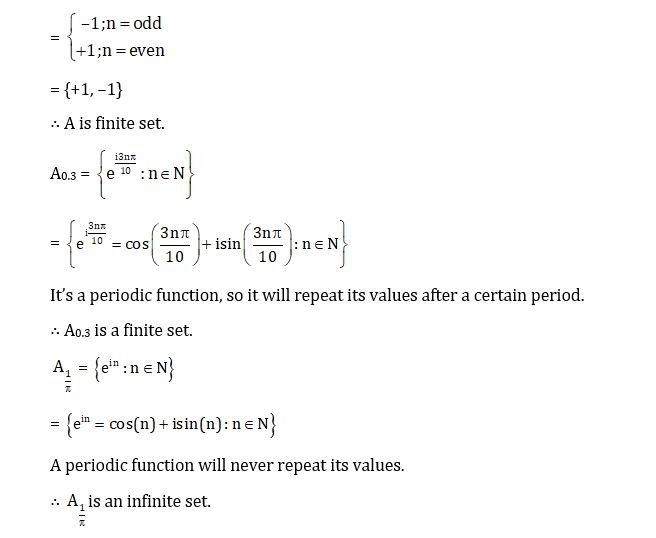Question 4. Number of integers k for which the equation x3 – 27x + k = 0 has at least two distinct integer roots is -

1. a) 1
2. b) 2
3. c) 3
4. d) 4

Solution:

Let, f(x) = x3–27x

⇒ f’(x) = 3 (x2–9) = 0

⇒ x = +3, –3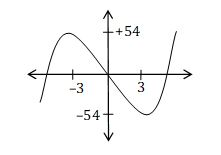Now, for f(x) = –k, to have at least two distinct roots k ∈ [–54, 54] …… (1)

Again, x3 – 27x + k = 0, has two distinct integral roots and sum of roots is zero. Therefore, third root has to be integer.

Let, the roots be α, β, –( α + β)

Also, ∑αβ = –27

⇒ α β + α (α + β) – β ( α + β) = –27

⇒ α2+ α β + β 2 = 27, α β ∈ I

∴ (α, β) ∈ {(–6,3), (–3–3), (–3,6), (3,–6), (3,3), (6,–3)} ..…. (2)

Again, ∏ α = –k

⇒ α . β (–(α + β)) = –k

⇒ α β (α + β) = k …… (3)

From eq. (1), (2) and (3)

The only possible values of ‘k’ are ±54.

Question 5. Suppose the tangent to the parabola y = x2 + px + q at (0, 3) has slope –1. Then p + q equals

1. a) 0
2. b) 1
3. c) 2
4. d) 3

Solution:

Given, y = x2 + px + q

Point (0, 3) lies on the curve.

∴ 3 = 0 + 0p + q

⇒ q = 3 …. (i)

Also, slope of tangent at (0,3) = –1

$\frac{dy}{dx}|_{(0,3)}$ = –1

⇒ 2(0) + p = –1

⇒ p = –1 ……(ii)

⇒ p + q = –1 + 3 = 2 {Using eq. (i) and (ii)}

Question 6. Let O = (0, 0); let A and B be points respectively on x-axis and y-axis such that

∠OBA = 60°. Let D be a point in the first quadrant such that OAD is an equilateral triangle. Then the slope of DB is –

1. a) √3
2. b) √2
3. c) 1/√2
4. d) 1/√3

Solution: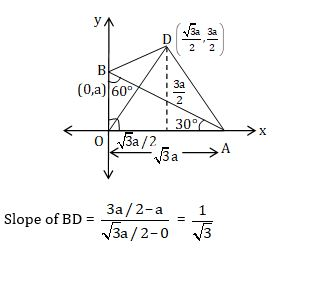Question 7. Suppose the parabola (y – k)2 = 4 (x – h), with vertex A, passes through O = (0, 0) and L = (0, 2). Let D be an end point of the latus rectum. Let the y-axis intersect the axis of the parabola at P. Then ∠PDA is equal to

1. a) $tan^{-1}\frac{1}{19}$
2. b) $tan^{-1}\frac{2}{19}$
3. c) $tan^{-1}\frac{4}{19}$
4. d) $tan^{-1}\frac{8}{19}$

Solution:

Given, (y–k)2 = 4(x–h)

As it passes through O (0,0) and L (0,2),

∴ (0–k)2 = 4(0–h); (2–k)2 = 4(0–h)

⇒ k2 = –4h; (2–k)2 = –4h

On solving, we get

k = 1, h = –1/4

∴ (y–1)2 = 4(x + 1/4) (Given Parabola)

⇒ Y2 = 4aX {where Y = y–1, X = x+1/4 and a = 1}

Ends of latus rectum: (a, ± 2a)

X = x +1/4 = 1 and Y = y–1 = ± 2

⇒ x = 3/4, y = 3, –1

$D\left ( \frac{3}{4}, 3 \right ) and\: D'\left ( \frac{3}{4}, -1 \right )$

Axis of parabola:

Y = y–1 = 0, which meets y-axis at (0,1)

∴ P (0,1)

Vertex: (0,0)

X = x + ¼ = 0, Y = y–1 = 0

x = –1/4, y = 1

∴ A (–1/4,1)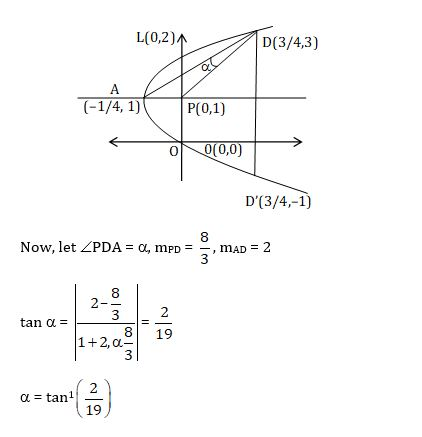Question 8. In a circle with center O, suppose A, P, B are three points on its circumference such that P is the mid-point of minor arc AB.

Suppose when $\angle AOB = \theta ,\frac{area(\Delta AOB)}{area(\Delta APB)} = \sqrt{5} + 2$.

If ∠AOB is doubled to 2θ, then the ratio $\frac{area(\Delta AOB)}{area(\Delta APB)}$ is:

1. a) $\frac{1}{\sqrt{5}}$
2. b) $\sqrt{5}-2$
3. c) $2\sqrt{3}+3$
4. d) $\frac{\sqrt{5-1}}{2}$

Solution:

Let, radius of circle be unity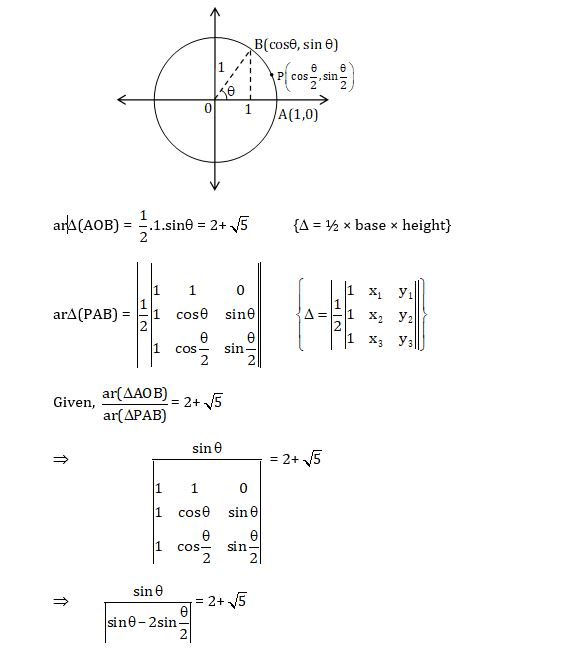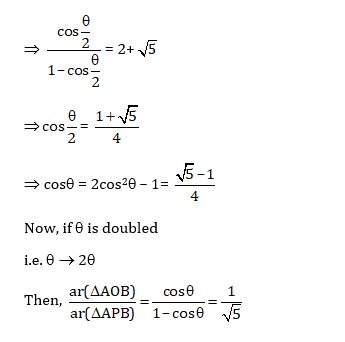Question 9. X = {x ∈ R: cos (sin x) = sin (cos x)}. The number of elements in X is:

1. a) 0
2. b) 2
3. c) 4
4. d) not finite

Solution:

X = {x ∈ R: cos (sin x) = sin (cos x)}

Now, cos (sinx) = sin (cosx)

$cos (sinx) = cos \left ( \frac{\pi }{2}-cos x \right )$

$sinx = 2n\pi \pm \left ( \frac{\pi }{2}-cos x \right ),$ n ∈ I

$sinx \mp cosx = 2n\pi \pm \frac{\pi }{2},$ n ∈ I

As L.H.S. ∈[-√2, √2]≠ R.H.S. for any n ∈ I

∴ No Solution.

Question 10. A sphere with center O sits atop a pole as shown in the figure. An observer on the ground is at a distance 50m from the foot of the pole. She notes that the angles of elevation from the observer to points P and Q on the sphere are 30° and 60°, respectively. Then, the radius of the sphere in meters is;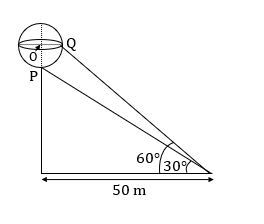1. a) $100\left (1 - \frac{1}{\sqrt{3}} \right )$
2. b) $\frac{50\sqrt{6}}{3}$
3. c) $50\left (1 - \frac{1}{\sqrt{3}} \right )$
4. d) $\frac{100\sqrt{6}}{3}$

Solution: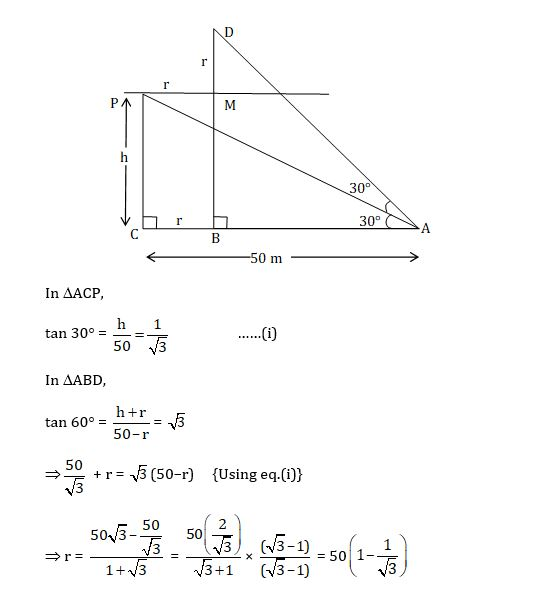Question 11. The graph of the function f(x) = x + 1/8 sin(2πx), 0 ≤ x ≤ 1 is shown below. Define

f1(x) = f(x), fn+1(x) = f(fn(x)), for n ≥ 1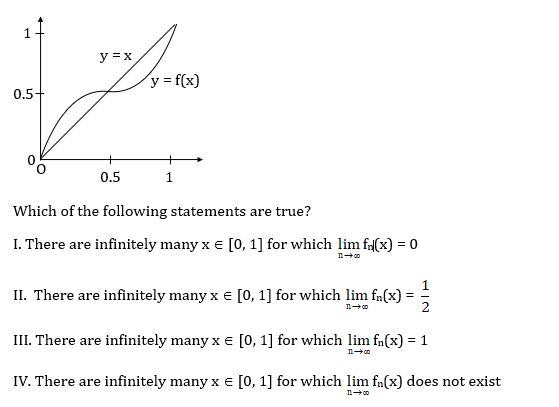1. a) I and III only
2. b) II only
3. c) I, II, III only
4. d) I, II, III and IV

Solution:

$\lim_{n\rightarrow \alpha }$fn(x) = f(f(f(.......infinity times(x))))

Now for x1 ∈ (0, 1/2)

f(x1) > x1 as f(x) is concave – downward

Thus, fn → 1/2 as n → ∞

Similarly, for x1 ∈ (1/2, 1)

f(x1) < x1 as f(x) is concave upward

Thus, fn → 1/2 as n → ∞

∴ There are infinitely many values of x in neighborhood of x = 1/2, for which

$\lim_{n\rightarrow \alpha }$fn(x) = 1/2

Question 12. The limit $\lim_{x\rightarrow \alpha }x^{2}\int_{0}^{x}e^{t^{3}-x^{3}}dt$ equals

1. a) 1/3
2. b) 2
3. c) ∞
4. d) 2/3

Solution: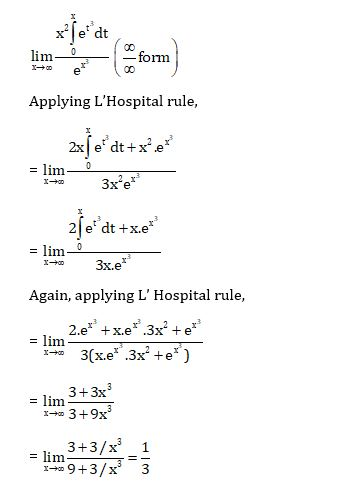Question 13. The polynomial equation x3 – 3ax2 + (27a2 + 9)x + 2016 = 0 has

1. a) exactly one real root for any real a
2. b) three real roots for any real a
3. c) three real roots for any a ≥ 0, and exactly one real root for any a < 0
4. d) three real roots for any a ≤ 0, and exactly one real root for any a > 0

Solution:

Given, f(x) = x3–3ax2 + (27a2+9) x + 2016

f’(x) = 3x2– 6ax + 27a2 + 9

= 3(x2–2ax+a2) + 24a2+9

= 3 (x–a)2 + 24a2 + 9 > 0

⇒ f(x) is strictly increasing odd degree polynomial.

∴ f(x) has only one real root.

Question 14. The area of the region bounded by the curve y = |x3 – 4x2 + 3x | and the x-axis, 0 ≤ x ≤ 3, is:

1. a) 37/6
2. b) 9/4
3. c) 37/12
4. d) 0

Solution: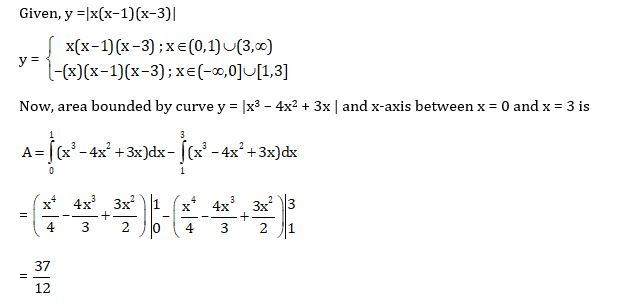Question 15. The number of continuous function f : [0, 1] → [0, 1] such that f(x) < x2 for all x and $\int_{0}^{1}f(x)dx = \frac{1}{3}$ is :

1. a) 0
2. b) 1
3. c) 2
4. d) infinite

Solution:

Given,

f: [0,1] → [0,1] and f(x) < x2

$\int_{0}^{1}f(x)dx < \int_{0}^{1}x^{2}dx$

$\int_{0}^{1}f(x)dx < \frac{1}{3}$

But, $\int_{0}^{1}f(x)dx = \frac{1}{3}$ (given)

∴ No such function exists.

Question 16. On the real line R, we define two functions f and g as follows:

f (x) = min{x – [x], 1 – x + [x]}

g (x) = max{x – [x], 1 – x + [x]}

Where [x] denotes the largest integer not exceeding x. The positive integer n for which$\int_{0}^{1}(g(x)-f(x))dx = 100$ is:

1. a) 100
2. b) 198
3. c) 200
4. d) 202

Solution: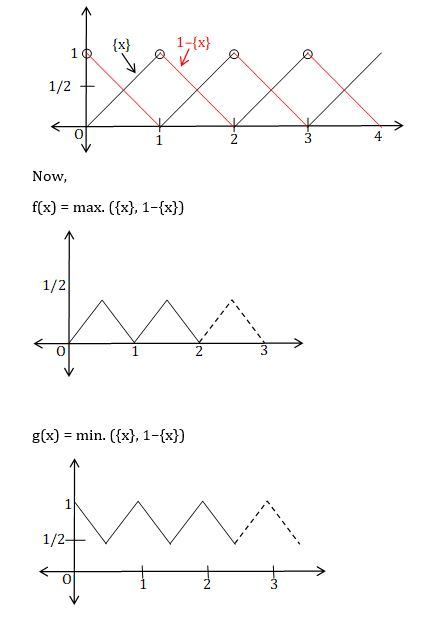Now, $\int_{0}^{1}g(x)-f(x)dx = 100$

$n\int_{0}^{1}g(x)-f(x)dx = 100$ {∵f(x) and g(x) are periodic functions with period 1}

$n\left ( \frac{3}{4} -\frac{1}{4}\right )= 100$

⇒ n = 200

Question 17. Let $\vec{v}$ be a vector in the plane such that $\left | \vec{v} - \vec{i} \right |$ = $\left | \vec{v} - \vec{2i} \right |$ = $\left | \vec{v} - \vec{j} \right |$. Then lies in the interval:

1. a) (0, 1]
2. b) (1, 2]
3. c) (2, 3]
4. d) (3, 4]

Solution:

Given, $\left | \vec{v} - \vec{i} \right |$ = $\left | \vec{v} - \vec{2i} \right |$ = $\left | \vec{v} - \vec{j} \right |$ ………. (i)

V $\vec{v}$ with position vector $\vec{v}$ is equidistant from $A(\hat{i}), B(\hat{2i})\: and\: C(\hat{j})$.

∴ V is Circum-Centre of ∆ABC.

Let, $\vec{v} = x\hat{i} + y\hat{j} \equiv (x,y)$

Again, |VA| = |VB| = |VC|

$\sqrt{(x-1)^{2}+y^{2}} = \sqrt{(x-2)^{2}+y^{2}} = \sqrt{x^{2}+(y-1)^{2}}$

⇒ (x, y) = (3/2, 3/2)

$\left | \vec{v} \right | = \frac{3}{2}(\hat{i}+\hat{j})$

$\left | \vec{v} \right | = \frac{3}{\sqrt{2}}\epsilon (2,3)$

Question 18. A box contains b blue balls and r red balls. A ball is drawn randomly from the box and is returned to the box with another ball of the same color. The probability that the second ball drawn from the box is blue is –

1. a) $\frac{b}{r+b}$
2. b) $\frac{b^{2}}{(r+b)^{2}}$
3. c) $\frac{b+1}{r+b+1}$
4. d) $\frac{b(b+1)}{(r+b)(r+b+1)}$

Solution: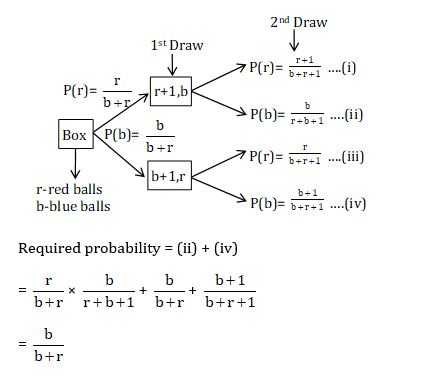Question 19. The number of non-congruent integer-sided triangles whose sides belong to the set {10, 11, 12, …., 22} is:

1. a) 283
2. b) 446
3. c) 448
4. d) 449

Solution:

The sides so picked must satisfy triangle in-equalities, i.e.

i) Sum of two sides > Third side

ii) Difference of two sides < Third side

Now,

No. of scalene triangles = 13C3 –3; {(10, 11, 21), (10, 11, 22), (10, 12, 22)}

= 283 .…. (1)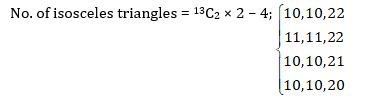= 152 ..… (2)

No. of equilateral triangles = 13C1 = 13 ….. (3)

From (1), (2) and (3)

∴Total no. of triangles = 448

Question 20. Suppose we have to cover the xy-plane with identical tiles such that no two tiles overlap and no gap is left between the tiles. Suppose that we can choose tiles of the following shapes; equilateral triangle, square, regular pentagon, regular hexagon. Then the tiling can be done with tiles of

1. a) all four shapes
2. b) exactly three of the four shapes
3. c) exactly two of the four shapes
4. d) exactly one of the four shapes

Solution:

If we are tiling a plane with congruent regular polygons, there must be n polygons meeting at each vertex. Thus, the interior angles of each polygon must be 2π/n, for some positive integer n.

For n=3, we get polygons with angles of 2π/3, which are regular hexagons. This tiling has three regular hexagons meeting at each vertex.

For n=4, we get polygons with angles of 2π/4 = π/2, which are squares. This tiling has four squares meeting at each vertex.

For n=5, the polygons would need to have angles of 2π/5. This is not possible for a regular polygon.

For n=6, the polygons would need to have angles of 2π/6 = π/3, which are equilateral triangles. This tiling has six triangles meeting at each vertex.

For n>6, the polygons would need to have angles less than π/3, which is impossible.

Question 21. The remainder when the polynomial 1 + x2 + x4 + x6 + …. + x22 is divided by 1 + x + x2 + x3 + …. + x11 is

1. a) 0
2. b) 2
3. c) 1 + x2 + x4 + …. + x10
4. d) 2(1 + x2 + x4 + …. + x10)

Solution: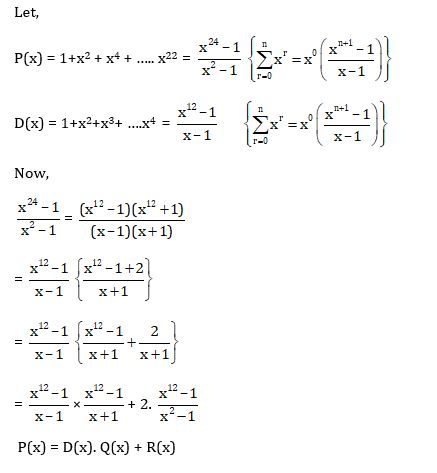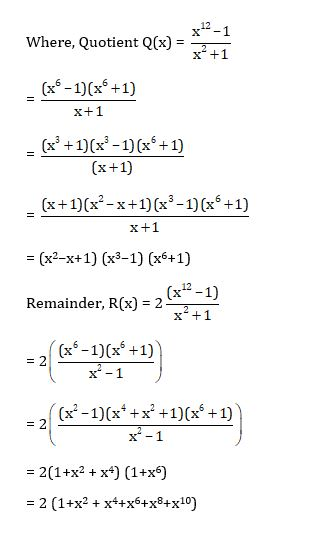Question 22. The range of the polynomial p(x) = 4x3 – 3x as x varies over the interval (-1/2, 1/2) is

1. a) [–1, 1]
2. b) (–1, 1]
3. c) (–1, 1)
4. d) (-1/2, 1/2)

Solution:

Given, p(x) = 4x3 – 3x

p’(x) = 12x2 – 3

= 3(4x2–1) < 0 ∀x ∈ (-1/2, 1/2)

⇒ p(x) is strictly decreasing function in (-1/2, 1/2)

⇒ Range of p(x) ∈((P1/2), P(–1/2) )

⇒ Range = (–1,1)

Question 23. Ten ants are on the real line. At time t = 0, the k-th ant starts at the point k2 and travelling at uniform speed, reaches the point (11 – k)2 at time t = 1. The number of distinct times at which at least two ants are at the same location is

1. a) 45
2. b) 11
3. c) 17
4. d) 9

Solution:

Velocity of kth ant = $\frac{(11-k)^{2}-k^{2}}{1}$ = 121–22k

Position of kth ant starting from k2,

xk = k2 + (121–22k) t

Now, let kth and pth ant meet after time ‘t’.

⇒ xk = xp

⇒ k2 + (121–22k) t = p2 + (121–22p) t

⇒ k2–p2 = 22t (k–p)

⇒ t = k + p/22

Here, k p ∈{1, 2,…..,10} and k ≠ p

∴ t ∈$\left \{ \frac{3}{22},\frac{4}{22}..............\frac{19}{22} \right \}$

i.e. 17 distinct values of ‘t’ at which at least two ants are at same position.

Question 24. A wall is inclined to the floor at an angle of 135°. A ladder of length ℓ is resting on the wall. As the ladder slides down, its mid-point traces an arc of an ellipse. Then the area of the ellipse is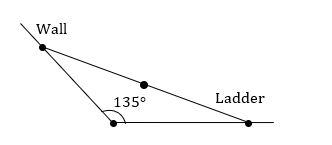1. a) πℓ2 / 4
2. b) πℓ2
3. c) 4πℓ2
4. d) 2πℓ2

Solution: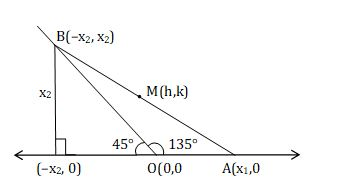Mid-point, M (h, k) = $\left ( \frac{x_{1}-x_{2}}{2},\frac{x_{2}}{2} \right )$

⇒ 2h = x1–x2; 2k = x2

⇒ x1= 2h + 2k, x2 = 2k

Also, AB = ℓ

⇒ AB2= ℓ2

⇒ (x1+x2)2 + x22 = ℓ2

⇒ (2h+4k)2 + (2k)2 + ℓ2

⇒ 4h2 + 20k2 + 15hk = ℓ2

$\frac{4x^{2}}{l^{2}}+\frac{16xy}{l^{2}}+\frac{20y^{2}}{l^{2}} = 1$ { ELLIPSE }

{Area of Ellipse, ax2 + bxy + cy2= 1 is $A = \frac{2\pi }{\sqrt{4ac-b^{2}}}$}

∴ Required area = $A = \frac{2{\pi l^{2}}}{\sqrt{320-256}} = \frac{\pi l^{2}}{4}$

Question 25. Let AB be a sector of a circle with center O and radius d, $\angle AOB = \theta \left ( < \frac{\pi }{2} \right )$, and D be a point on OA such that BD is perpendicular OA. Let E be the midpoint of BD and F be a point on the arc AB such that EF is parallel to OA. Then the ratio of length of the arc AF to the length of the arc AB is;

1. a) 1/2
2. b) θ/2
3. c) $\frac{1}{2}sin\theta$
4. d) $\frac{sin^{-1}\left ( \frac{1}{2}sin\theta \right )}{\theta}$

Solution: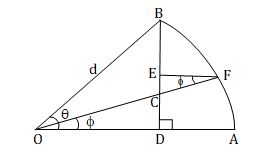In ∆OBD,

OD = d cos θ; BD = d sinθ, ED = ½ BD = ½ d sinθ …….(1)

In ∆OCD,

OC = OD sec ϕ d cos θ sec ϕ, CD = OD tan ϕ = d cos θ tan ϕ …. (2)

In ∆CEF,

EC = CF sinϕ

= (OF – OC) sinϕ

= (d – d cos θ sec ϕ) sinϕ {Using (2)}

= d (sinϕ – cos θ tan ϕ) ……(3)

Also, ED = EC + CD {using (1), (2) and (3)}

$\frac{dsin\theta }{2}$ = d(sin ϕ –cosθ tan ϕ) + d cosθ. tanϕ

$\frac{sin\theta }{2}$ = sin ϕ

⇒ ϕ = sin–1$\left ( \frac{sin\theta }{2} \right )$

Now, $\frac{arc(AF)}{arc(AB)} = \frac{\phi }{\theta } = \frac{sin^{-1}(\frac{sin\theta}{2})}{\theta }$

Question 26. Let f(x) be a non-negative differentiable function on [0, ∞) such that f '(0) = 0 and f ' (x) ≤ 2f(x) for all x > 0. Then, on [0, ∞)

1. a) f(x) is always a constant function
2. b) f(x) is strictly increasing
3. c) f(x) is strictly decreasing
4. d) f '(x) changes sign

Solution:

Given, f’(x) ≤ 2f(x) ∀ x ≥ 0

Multiplying both sides by e–2x

⇒ e–2x f’(x) ≤ 2e–2x f(x)

⇒ e–2x f’(x) – 2e–2x f(x) ≤ 0

⇒ d/dx (e–2xf(x)) ≤ 0

⇒ g(x) = e–2xf(x) is decreasing function ∀ x ≥ 0

⇒ g(0) ≥ g(x) ; x ≥ 0

⇒ e–0f(0) ≥ e–2xf(x) ; x ≥ 0

⇒ f(x) e–2x ≤ 0 ; x ≥ 0 {∵ f(0)=0 }

⇒ f(x) ≤ 0 ; x ≥ 0 {e–2x ≠0}

Also, f(x) ≥ 0 ∀ x ≥ 0 {given}

∴ f(x) = 0 ∀ x ≥ 0

A constant function.

Question 27. For each positive real number λ, let Aλ be the set of all-natural numbers n such that |sin√(n+1) – sin √n| < λ. Let Aλc be the complement of Aλ in the set of all-natural numbers. Then -

1. a) A1/2, A1/3, A2/5 are all finite sets
2. b) A1/3 is a finite set but A1/2, A2/5 are infinite sets
3. c) A1/2c, A1/3c, A2/5c are all finite sets
4. d)A1/3, A2/5 are finite sets and A1/2 is an infinite set

Solution:

As n →∞, |sin √(n+1) –sin (√n)| → 0

⇒ There exist infinite natural numbers ‘n’ such that:

|sin √(n+1) –sin (√n)| <λ, ∀ λ > 0

∴ A1/2, A1/3, A2/5 are all infinite sets.

Question 28. Let f be a continuous function defined on [0, 1] such that $\int_{0}^{1}f^{2}(x)dx = \left ( \int_{0}^{1}f(x)dx \right )^{2}$. Then the range of f

1. a) has exactly two points
2. b) has more than two points
3. c) is the interval [0, 1]
4. d) is a singleton

Solution: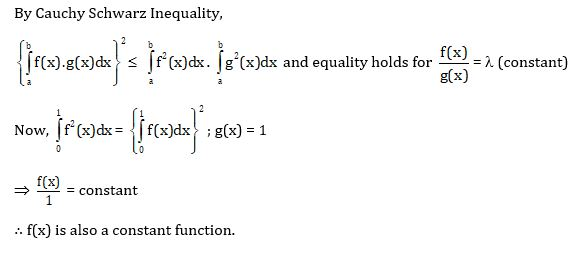Question 29. Three schools send 2, 4 and 6 students, respectively, to a summer camp. The 12 students must be accommodated in 6 rooms numbered 1, 2, 3, 4, 5, 6 in such a way that each room has exactly 2 students and both from the same school. The number of ways, the students can be accommodated in the rooms is -

1. a) 60
2. b) 45
3. c) 32400
4. d) 2700

Solution: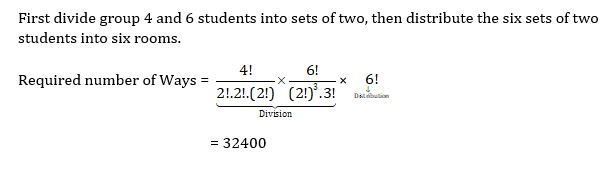Question 30. Let a be a fixed non-zero complex number with |a| < 1 and ω = $\left ( \frac{z-a}{1-\bar{a}z} \right )$. Where z is a complex number. Then

1. a) there exists a complex number z with |z| < 1 such that |ω| > 1
2. b) |ω| > 1 for all z such that |z| < 1
3. c) |ω| < 1 for all z such that |z| < 1
4. d) there exists z such with |z| < 1 and |ω| = 1

Solution: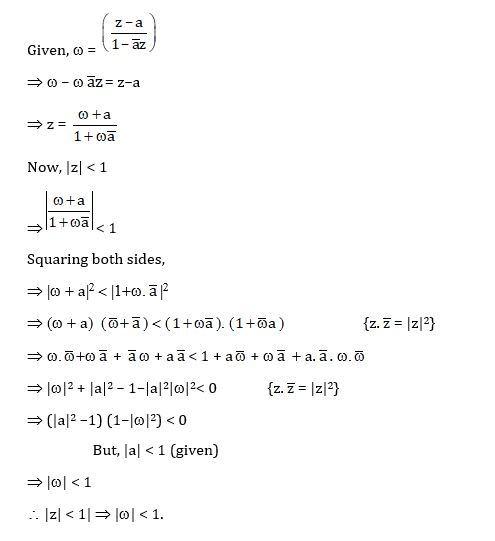### KVPY SX 2016 Maths Paper with Solutions